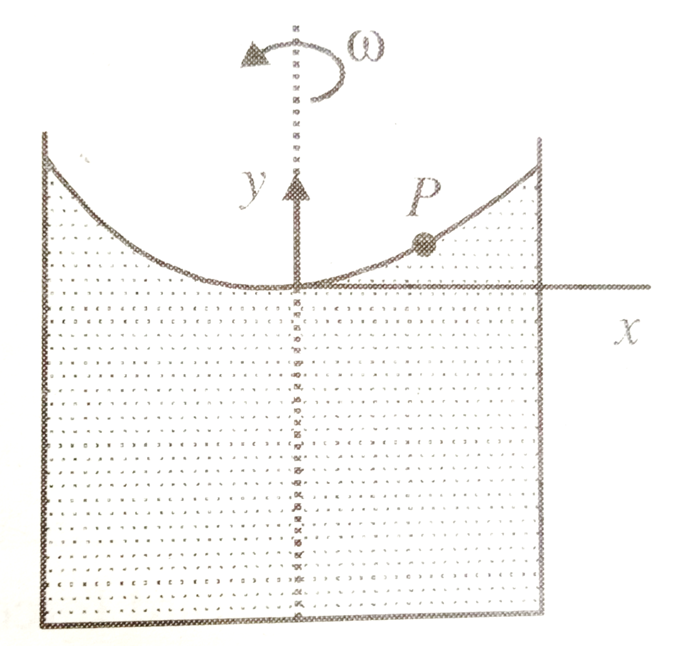Consider a liquid of density $\rho$ in a container that spins with angular velocity $\omega$ as shown in figure. Find relation between y and x for any point P, if liquid rises due to rotation. [This question is only for Dropper and XII batch]1. $y=\frac{\omega x}{2g}$

2. $y=\frac{{\omega }^{2}{x}^{2}}{2g}$

3. $y=\frac{{\omega }^{2}x}{2g}$

4. $y=\frac{\omega {x}^{2}}{2g}$

Concept Questions :-

Integration
High Yielding Test Series + Question Bank - NEET 2020

Difficulty Level:

The upper edge of a gate in a dam runs along water surface. The gate is 2 m high and 3 m wide and is hinged along a horizontal line through its center. Calculate the torque about hinge. [This question is only for Dropper and XII batch]

1.

2.

3.

4.

Concept Questions :-

Integration
High Yielding Test Series + Question Bank - NEET 2020

Difficulty Level:

The specific heat of a substance varies with temperature t($°C$) as
c= 0.20 + 0.14 t + 0.023 . Find the heat required to raise the temperature of 2 gm of substance from 5$°C$ to 15 $°C$[This question is only for Dropper and XII batch]

1. 41 cal

2. 82 cal

3. 80 cal

4. 40 cal

High Yielding Test Series + Question Bank - NEET 2020

Difficulty Level:

An isolated container at 127$°C$ contains an ice cube of mass 100 g at 0$°C$. The specific heat C of container varies with temperature according to relation C= a+bT, where a= 0.1 kcal/kg-K and b= 40 m cal/kg K. Find the mass of container, if the final temperature of container is 300 K.
[Take ${L}_{F}$= 80 cal/g and specific heat of water 1 cal/g K]

[This question is only for Dropper and XII batch]

1. 500 g

2. 720 g

3. 940 g

4. 1200 g

Concept Questions :-

Integration
High Yielding Test Series + Question Bank - NEET 2020

Difficulty Level:

The temperature of n moles of an ideal gas is increased from  through a process $P=\frac{\alpha }{T}$ . Find the work done by the gas. [This question is only for Dropper and XII batch]

1. $\frac{nR{T}_{0}}{2}$

2. $nR{T}_{0}$

3. $\frac{3}{2}nR{T}_{0}$

4. 2 $nR{T}_{0}$

Concept Questions :-

Integration
High Yielding Test Series + Question Bank - NEET 2020

Difficulty Level:

Electric field is given by E $=\frac{100}{{x}^{2}}$ . Find the potential difference between x= 10 and x= 20 m. [This question is only for Dropper and XII batch]

1. 5 V

2. 10 V

3. 15 V

4. 20 V

Concept Questions :-

Integration
High Yielding Test Series + Question Bank - NEET 2020

Difficulty Level:

Calculate the electric field on the axis of a very long uniformly charged, thin rod at a distance r from one end. The charge per unit length of the rod is $\lambda$[This question is only for Dropper and XII batch]

1. $\frac{2k\lambda }{r}$

2. $\frac{k\lambda }{r}$

3. $\frac{k\lambda }{2r}$

4. $\frac{k\lambda }{4r}$

High Yielding Test Series + Question Bank - NEET 2020

Difficulty Level:

Find electric field at the centre of arc which subtends '$\theta$' angle at centre

[This question is only for Dropper and XII batch]

1.

2. $\frac{2k\lambda }{r}\mathrm{sin}\frac{\theta }{4}$

3. $\frac{2k\lambda }{r}\mathrm{sin}\frac{\theta }{2}$

4. $\frac{2k\lambda }{r}\mathrm{sin}2\theta$

Concept Questions :-

Integration
High Yielding Test Series + Question Bank - NEET 2020

Difficulty Level:

Find electric field due to a uniformly charged, long and thin rod

[This question is only for Dropper and XII batch]

1. $\frac{k\lambda }{r}$

2. $\frac{k\lambda }{2r}$

3. $\frac{2k\lambda }{r}$

4. $\frac{k\lambda }{4r}$

Concept Questions :-

Integration
High Yielding Test Series + Question Bank - NEET 2020

Difficulty Level:

A ball of radius R carries a positive charge throughout its volume, such that the volume density of charge depends on distance r from the ball's centre as $\rho ={\rho }_{0}\left(1-\frac{r}{R}\right)$, where ${\rho }_{0}$ is a constant. Assuming the permittivity of ball to be one, find magnitude of electric field as a function of distance r, both inside  the ball. [This question is only for Dropper and XII batch]

1.$\frac{{\rho }_{0}r}{3{\epsilon }_{0}}$

2. $\frac{{\rho }_{0}\left(4rR-3{r}^{2}\right)}{12{\epsilon }_{0}R}$

3. $\frac{{\rho }_{0}\left(4rR-3{r}^{2}\right)}{3{\epsilon }_{0}R}$

4. $\frac{{\rho }_{0}\left(4rR-5{r}^{2}\right)}{3{\epsilon }_{0}R}$

Concept Questions :-

Integration